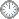# “机甲大战”的证明(b++)

This is an in-mail from TYUST.

新入の者--> What is going on ? (redirected)new

(接前: 03 01 29) “机甲大战” (Pro5.9) 的证明.(Step2的概念化·续)

.

Step1 准备了个  变配对 (X, U + vU) eps'/2-lc, 但 Step2 是以 控制配对 (X, U) eps'-lc 作为主导 ——

.

W   ~  T

↓

X        U

.

.

ψ 的超常除子.

---- ψ 是相对于 控制配对 (X, U) 而言.

---- ψ 的超常除子记作 Ei 及 T.

---- 单独标识 T 是因其特殊性.

---- Ei 和 T 都在 W 上 (on).

.

隙函数的取值.

---- a = a(···) 可看做三元泛函.

---- 第一个“·” 填入素除子.

---- 后两个“·” 填入配对.

1) 用控制配对(X, U) 引导, 则有:

---- ai = a(Ei, X, U).

----  a = a( T, X, U). 注意: 按条件恰好 a = eps'.

2) 用变配对(X, U + vU) 引导, 则有:

---- ai' = a(Ei, X, U + vU).

----  a' = a( T, X, U + vU).

.

---- U + vU = (1 + v)U, 仅和 U 差个因子(1 + v).

---- 或许, 这回答了前述疑问(?).

.

回到 Step2.

1. 给出变形的全像:

Γw = (1 + v)U~ + (1 - eps'/4)ΣEi + (1 - eps')T.

.

2. 用控制配对引导锻公式.

---- 从 Kw + Γw 出发, 凑出锻公式:

Kw + Γw = Kw + (1 + v)U~ + (1 - eps'/4)ΣEi + (1 - eps')T

= Kw +U~ + Σ(1 - ci)Ei + (1 - eps')T + Σ(ci - eps'/4)Ei

= ψ*(Kx + U) + vU~ +  F

.

.

3. 用变配对引导锻公式.

---- 从 Kw + Γw 出发, 凑出锻公式:

Kw + Γw = Kw + (1 + v)U~ + (1 - eps'/4)ΣEi + (1 - eps')T

= Kw + (1 + v)U~ +Σ(1 - ci')Ei + (1 - a')T + Σ(ci' - eps'/4)Ei + (a' - eps')T

ψ*(Kx + (1 + v)U) + G

.

.

---- 要重新斟酌 “变配对” 这个名称.

---- “变像” 是 (1+v)U的本像, 相对于U而言“变”了.

---- 锻公式“变形”是指出现了余项, 这是由 Γw 的构造引起的(与变配对无关).

.

符号大全上下标.|| 常用：↑↓ πΓΔΛΘΩμφΣ &Oslash; ∈ ∉ ∪ ∩ ⊆ ⊇ ⊂ ⊃ ≤ ≥ ⌊ ⌋ ⌈ ⌉ ≠ ≡ ⁰ &sup1; &sup2; &sup3; ᵈ ₀ ₁ ₂ ₃ ᵢ .

#### Glossary(AG)

*

Abstract 8/4

Introduction

....

....

.Proposition 5.2 11/9

...

Proposition 5.5. 11/5

http://blog.sciencenet.cn/blog-315774-1204885.html

## 全部精选博文导读

GMT+8, 2020-2-21 05:39